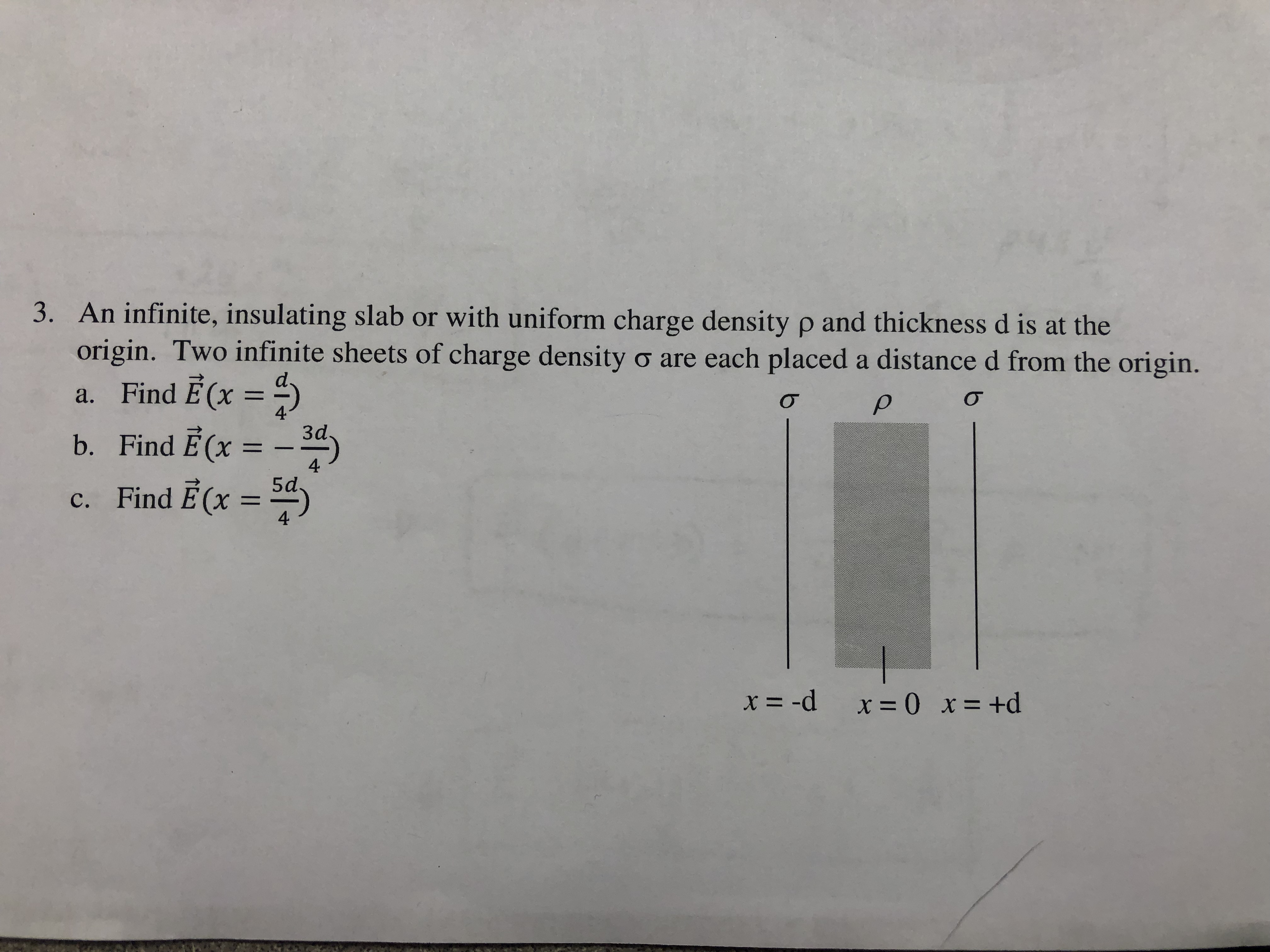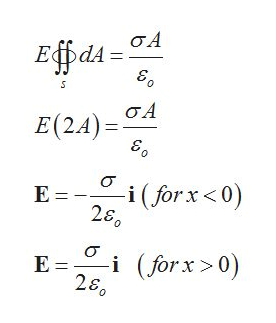# 3. An infinite, insulating slab or with uniform charge density p and thickness d is at theorigin. Two infinite sheets of charge density o are each placed a distance d from the origin.a. Find E(x )σ3db. Find E (x425dc. Find E(x114x= -dx =0 x= +d

Question
87 viewshelp_outlineImage Transcriptionclose3. An infinite, insulating slab or with uniform charge density p and thickness d is at the origin. Two infinite sheets of charge density o are each placed a distance d from the origin. a. Find E(x ) σ 3d b. Find E (x 42 5d c. Find E(x 11 4 x= -d x =0 x= +d fullscreen
check_circle

star
star
star
star
star
2 Ratings
Step 1

The expression for the Electric field intensity due to infinite sheet of charge is calculated from the integral form of the Gauss’s Law for electrostatics.

Step 2

Here qenc is the charge enclosed by the sheet, E is the electric field vector, dA is the differential area vector, εo is the permittivity of free space.

Given the surface charge density σ of the infinite sheet of charge the, charge enclosed will be,

Step 3

For an infinite sheet of charge the electric field line and the area vector are parallel to each other, and the field lines point in both direction away from...help_outlineImage TranscriptioncloseЕ фал- ОА Е(24) - 9A о3 i (forx<0) E =- E =i(forx>0) 280 fullscreen

### Want to see the full answer?

See Solution

#### Want to see this answer and more?

Solutions are written by subject experts who are available 24/7. Questions are typically answered within 1 hour.*

See Solution
*Response times may vary by subject and question.
Tagged in

### Science